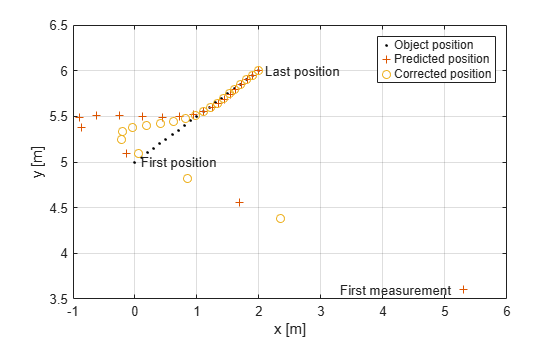Main Content

# predict

Predict state and state estimation error covariance of linear Kalman filter

## Syntax

``[xpred,Ppred] = predict(filter)``
``[xpred,Ppred] = predict(filter,u)``
``[xpred,Ppred] = predict(filter,F)``
``[xpred,Ppred] = predict(filter,F,Q)``
``[xpred,Ppred] = predict(filter,u,F,G)``
``[xpred,Ppred] = predict(filter,u,F,G,Q)``
``[xpred,Ppred] = predict(filter,dt)``
``[xpred,Ppred] = predict(filter,u,dt)``
``predict(filter,___)``
``xpred = predict(filter,___)``

## Description

````[xpred,Ppred] = predict(filter)` returns the predicted state, `xpred`, and the predicted state estimation error covariance, `Ppred`, for the next time step of the input linear Kalman filter. The predicted values overwrite the internal state and state estimation error covariance of `filter`.This syntax applies when you set the `ControlModel` property of `filter` to an empty matrix.```
````[xpred,Ppred] = predict(filter,u)` specifies a control input, or force, `u`, and returns one or more of the outputs from the preceding syntaxes.This syntax applies when you set the `ControlModel` property of `filter` to a nonempty matrix.```
````[xpred,Ppred] = predict(filter,F)` specifies the state transition model, `F`. Use this syntax to change the state transition model during a simulation.This syntax applies when you set the `ControlModel` property of `filter` to an empty matrix.```
````[xpred,Ppred] = predict(filter,F,Q)` specifies the state transition model, `F`, and the process noise covariance, `Q`. Use this syntax to change the state transition model and process noise covariance during a simulation.This syntax applies when you set the `ControlModel` property of `filter` to an empty matrix.```
````[xpred,Ppred] = predict(filter,u,F,G)` specifies the force or control input, `u`, the state transition model, `F`, and the control model, `G`. Use this syntax to change the state transition model and control model during a simulation.This syntax applies when you set the `ControlModel` property of `filter` to a nonempty matrix.```
````[xpred,Ppred] = predict(filter,u,F,G,Q)` specifies the force or control input, `u`, the state transition model, `F`, the control model, `G`, and the process noise covariance, `Q`. Use this syntax to change the state transition model, control model, and process noise covariance during a simulation.This syntax applies when you set the `ControlModel` property of `filter` to a nonempty matrix.```

example

````[xpred,Ppred] = predict(filter,dt)` returns the predicted outputs after time step `dt`.This syntax applies when the `MotionModel` property of `filter` is not set to `'Custom'` and the `ControlModel` property is set to an empty matrix.```
````[xpred,Ppred] = predict(filter,u,dt)` also specifies a force or control input, `u`.This syntax applies when the `MotionModel` property of `filter` is not set to `'Custom'` and the `ControlModel` property is set to a nonempty matrix.```
````predict(filter,___)` updates `filter` with the predicted state and state estimation error covariance without returning the predicted values. Specify the tracking filter and any of the input argument combinations from preceding syntaxes.```
````xpred = predict(filter,___)` updates `filter` with the predicted state and state estimation error covariance but returns only the predicted state, `xpred`.```

## Examples

collapse all

Create a linear Kalman filter that uses a `2D Constant Velocity` motion model. Assume that the measurement consists of the object's x-y location.

Specify the initial state estimate to have zero velocity.

```x = 5.3; y = 3.6; initialState = [x;0;y;0]; KF = trackingKF('MotionModel','2D Constant Velocity','State',initialState);```

Create the measured positions from a constant-velocity trajectory.

```vx = 0.2; vy = 0.1; T = 0.5; pos = [0:vx*T:2;5:vy*T:6]';```

Predict and correct the state of the object.

```for k = 1:size(pos,1) pstates(k,:) = predict(KF,T); cstates(k,:) = correct(KF,pos(k,:)); end```

Plot the tracks.

```plot(pos(:,1),pos(:,2),'k.', pstates(:,1),pstates(:,3),'+', ... cstates(:,1),cstates(:,3),'o') xlabel('x [m]') ylabel('y [m]') grid xt = [x-2 pos(1,1)+0.1 pos(end,1)+0.1]; yt = [y pos(1,2) pos(end,2)]; text(xt,yt,{'First measurement','First position','Last position'}) legend('Object position', 'Predicted position', 'Corrected position')```## Input Arguments

collapse all

Linear Kalman filter for object tracking, specified as a `trackingKF` object.

Control vector, specified as a real-valued L-element vector.

State transition model, specified as a real-valued M-by-M matrix, where M is the size of the state vector.

Process noise covariance matrix, specified as a positive-definite, real-valued M-by-M matrix, where M is the length of the state vector.

Control model, specified as a real-valued M-by-L matrix. M is the size of the state vector. L is the number of independent controls.

Time step, specified as a positive scalar. Units are in seconds.

## Output Arguments

collapse all

Predicted state, returned as a real-valued M-element vector. The predicted state represents the deducible estimate of the state vector, propagated from the previous state using the state transition and control models.

Predicted state covariance matrix, specified as a real-valued M-by-M matrix. M is the size of the state vector. The predicted state covariance matrix represents the deducible estimate of the covariance matrix vector. The filter propagates the covariance matrix from the previous estimate.

## See Also

Introduced in R2017a

## Support

#### Implementing an Adaptive Cruise Controller with Simulink

Download technical paper